# ISEE Upper Level Quantitative : How to find mean

## Example Questions

← Previous 1 3 4

### Example Question #1 : How To Find Mean

This semester, Mary had five quizzes that were each worth 10% of her grade. She scored 89, 74, 84, 92, and 90 on those five quizzes. Mary also scored a 92 on her midterm that was worth 25% of her grade, and a 91 on her final that was also worth 25% of her class grade. What was Mary's final grade in the class?

89

85

87

91

89

Explanation:

To find her average grade for the class, we need to multiply Mary's test scores by their corresponding weights and then add them up.

The five quizzes were each worth 10%, or 0.1, of her grade, and the midterm and final were both worth 25%, or 0.25.

average = (0.1 * 89) + (0.1 * 74) + (0.1 * 84) + (0.1 * 92) + (0.1 * 90) + (0.25 * 92) + (0.25 * 91) = 88.95 = 89.

Looking at the answer choices, they are all spaced 2 percentage points apart, so clearly the closest answer choice to 88.95 is 89.

### Example Question #2 : How To Find Mean

Consider the following data set: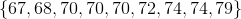Which of these numbers is greater than the others?

It cannot be determined from the information given

The midrange of the set

The mean of the set

The mode of the set

The median of the set

The midrange of the set

Explanation:

The median of the set is the fifth-highest value, which is 70; this is also the mode, being the most commonly occurring element.

The mean is the sum of the elements divided by the number of them. This is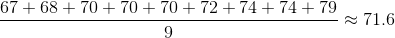The midrange is the mean of the least and greatest elements, This is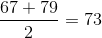The midrange is the greatest of the four.

### Example Question #3 : How To Find Mean

Which is true about the mean and the median of the following data set: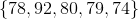The median exceeds the mean by less than 5.

The mean exceeds the median by 5 or more.

The mean exceeds the median by less than 5.

The median exceeds the mean by 5 or more.

The median and the mean are equal.

The mean exceeds the median by less than 5.

Explanation:

The mean of the data set is the sum of the elements divided by 5: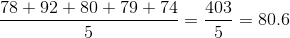The median of the data set is the middle value when the values are arranged in ascending order, which here is the third-highest value. This is 79.

The mean exceeds the median by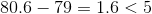### Example Question #4 : Mean

Mark's numeric grade in his Spanish class is determined by five equally weighted hourly tests and a final, weighted twice as much as an hourly test. The highest score possible on each is 100.

Going into finals week, Mark's hourly test scores are 92, 66, 84, 77, and 87. What must Mark score on his final, at minimum, in order to achieve a grade of 80 or better for the term?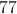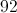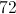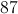Explanation:

Mark's grade is a weighted mean in which his hourly tests have weight 1 and his final has weight 2. If we callhis final, then his term average will be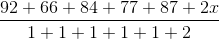,

which simplifies to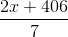.

Since Mark wants his score to be 80 or better, we solve this inequality: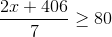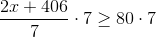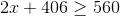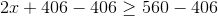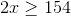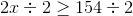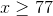Mark must score 77 or better on his final.

### Example Question #5 : Mean

A gymnastics contest has seven judges, each of whom rates each contestant's performance on a scale from 0 to 10. A contestant's score is calculated by disregarding the highest and lowest scores, and taking the mean of the remaining five scores.

The seven judges rated Sally's performance with the following seven scores: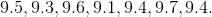They rated Sue's performance with the following seven scores: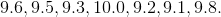Which of these quantities is the greater?

(a) Sally's score

(b) Sue's score

(a) is greater.

It cannot be determined from the information given.

(a) and (b) are equal.

(b) is greater.

(b) is greater.

Explanation:

To calculate whether Sally or Sue has the higher average, it is only necessary to add, for each contestant, all of their scores except for their highest and lowest. Since both sums are divided by 5, the higher sum will result in the higher mean score.

(a) For Sally, the highest and lowest scores are 9.7 and 9.1. The sum of the other five scores is: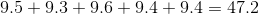(b) For Sue, the highest and lowest scores are 10.0 and 9.1. The sum of the other five scores is: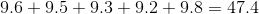Sue's total - and, subsequently, her score - is higher than Sally's, so (b) is the greater quantity.

### Example Question #4 : How To Find Mean

A gymnastics meet has seven judges. After each routine, each judge assigns a merit-based score from 0 to 10; a contestant's score for the routine is the mean of all the judges' scores except for the highest and the lowest.

The seven judges individually assigned the following scores to one of Kathy's routines: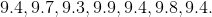Which is the greater quantity?

(a) Kathy's score for the routine

(b) 9.5

(a) and (b) are equal.

(b) is greater.

It is impossible to tell from the information given.

(a) is greater.

(a) is greater.

Explanation:

The highest and lowest scores of the seven are 9.9 and 9.3, so Kathy's score is the mean of the other five: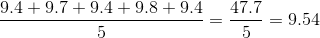This makes (a) greater.

### Example Question #585 : Isee Upper Level (Grades 9 12) Quantitative Reasoning

A student's course average is determined by calculating the mean of five tests. Chuck is trying for an average ofin the course; his first four test scores are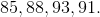Which is the greater quantity?

(a) The score Chuck needs on the fifth test to achieve his goal

(b)(a) and (b) are equal.

It is impossible to tell from the information given.

(a) is greater.

(b) is greater.

(a) is greater.

Explanation:

For Chuck to achieve an average of, his scores on the five tests must total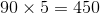. At current, his scores total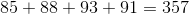, so he needs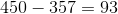points to achieve his average. This makes (a) greater.

### Example Question #8 : Mean

Which is the greater quantity?

(a) The mean of the data set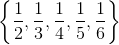(b)(a) is greater

(a) and (b) are equal

It is impossible to tell from the information given

(b) is greater

(a) is greater

Explanation:

The sum of the elements in the data setis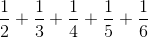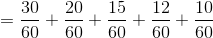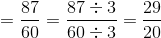Divide by 5 to get the mean: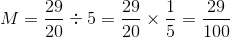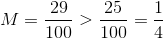### Example Question #9 : Mean

Which is the greater quantity?

(a) The mean of the data set: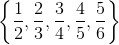(b)(b) is greater.

It is impossible to tell from the information given.

(a) and (b) are equal.

(a) is greater.

(b) is greater.

Explanation:

The sum of the elements in the data setis: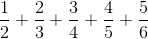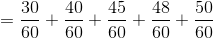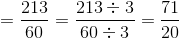Divide by 5 to get the mean: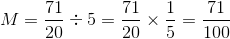(b)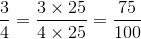(b) is greater.

### Example Question #5 : How To Find Mean

Which is the greater quantity?

(a) The mean of the data set: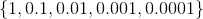(b)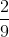(a) and (b) are equal.

It is impossible to tell from the information given.

(a) is greater.

(b) is greater.

(b) is greater.

Explanation:

(a) The mean of the data set is the sum of its elements divided by: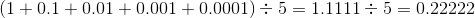(b)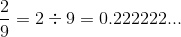(b) is greater.

← Previous 1 3 4